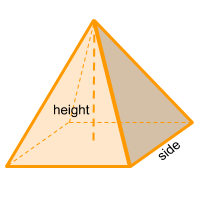Home » Polyhedra » Pyramids » Pyramid

# Volume of a PyramidVolume =
√2 × Side³/6

## Volume calculator for a pyramid

Enter the side of the square (Side) that forms the base of the pyramid to get the volume automatically.

## Description, how many faces, edges and vertices are there in a pyramid

The pyramid or square pyramid, has 5 faces, the 4 faces of the sides are triangles and the base is a square. It has 5 vertices and 8 edges.

## Examples of a pyramid

We can find many objects that look like a pyramid, examples of pyramid shaped objects are: pyramids of Egypt or the Louvre museum pyramid in France. Can you think of any other examples? leave a comment in the comment section at the bottom of the page.

## Formula for the volume of a pyramid

In case the base of the pyramid is rectangular and not square, then we are in the presence of a rectangular pyramid, in which case we need to calculate its base according to the area formula of a rectangle.

To calculate the volume of a square pyramid is very simple, we only have to take one side of the square base of the pyramid and square it, then multiply it by the height of the pyramid and finally divide the result by 3. Also you can use the online calculator to get the volume of the pyramid automatically.

Volume =
√2 × Side³/6

Formula explanation:

The formula to calculate the volume of a pyramid is very simple:

Volume =
Height × Side² /3

The formula to calculate the height of a pyramid is:

Height =
1 / √2
× side

Then you can replace the height value in the formula:

Volume =
√(½) × Side³ /3
=
2 × √(½) × Side³ /2 × 3
=
√2 × Side³ /6

Share